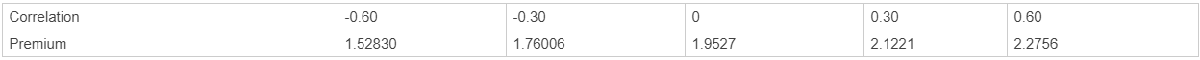Price European basket options using Nengjiu Ju approximation model

example

Examples

collapse all

Find a European call basket option of two stocks. Assume that the stocks are currently trading at \$10 and \$11.50 with annual volatilities of 20% and 25%, respectively. The basket contains one unit of the first stock and one unit of the second stock. The correlation between the assets is 30%. On January 1, 2009, an investor wants to buy a 1-year call option with a strike price of \$21.50. The current annualized, continuously compounded interest rate is 5%. Use this data to compute the price of the call basket option with the Nengjiu Ju approximation model.

Settle = 'Jan-1-2009';
Maturity  = 'Jan-1-2010';

% Define the RateSpec.
Rate = 0.05;
Compounding = -1;
RateSpec = intenvset('ValuationDate', Settle, 'StartDates', ...
Settle, 'EndDates', Maturity, 'Rates', Rate, 'Compounding', Compounding);

% Define the Correlation matrix. Correlation matrices are symmetric and
% have ones along the main diagonal.
Corr = [1 0.30; 0.30 1];

AssetPrice =  [10;11.50];
Volatility = [0.2;0.25];
Quantity = [1;1];

% Compute the price of the call basket option.
OptSpec = {'call'};
Strike = 21.5;
PriceCorr30 = 2.1221

Compute the price of the basket instrument for these two stocks with a correlation of 60%. Then compare this cost to the total cost of buying two individual call options.

Corr = [1 0.60; 0.60 1];

% Compute the price of the call basket option with Correlation = -0.60
PriceCorr60 = 2.2757

The following table summarizes the sensitivity of the option to correlation changes. In general, the premium of the basket option decreases with lower correlation and increases with higher correlation.Compute the cost of two vanilla 1-year call options using the Black-Scholes (BLS) model on the individual assets:

StockSpec = stockspec(Volatility, AssetPrice);
StrikeVanilla= [10;11.5];

PriceVanillaOption = optstockbybls(RateSpec, StockSpec, Settle, Maturity,...
OptSpec, StrikeVanilla)
PriceVanillaOption = 2×1

1.0451
1.4186

Find the total cost of buying two individual call options.

sum(PriceVanillaOption)
ans = 2.4637

The total cost of purchasing two individual call options is \$2.4637, compared to the maximum cost of the basket option of \$2.27 with a correlation of 60%.

Input Arguments

collapse all

Interest-rate term structure (annualized and continuously compounded), specified by the RateSpec obtained from intenvset. For information on the interest-rate specification, see intenvset.

Data Types: struct

Data Types: struct

Definition of the option as 'call' or 'put', specified as a character vector or a 2-by-1 cell array of character vectors.

Data Types: char | cell

Option strike price value, specified as one of the following:

• For a European or Bermuda option, Strike is a scalar (European) or 1-by-NSTRIKES (Bermuda) vector of strike prices.

• For an American option, Strike is a scalar vector of the strike price.

Data Types: double

Settlement or trade date for the basket option, specified as a scalar serial date number or date character vector.

Data Types: double | char

Maturity date for the basket option, specified as a scalar serial date number or date character vector.

Data Types: double | char

Output Arguments

collapse all

Expected price for basket option, returned as a numeric.

collapse all

A basket option is an option on a portfolio of several underlying equity assets.

Payout for a basket option depends on the cumulative performance of the collection of the individual assets. A basket option tends to be cheaper than the corresponding portfolio of plain vanilla options for these reasons:

• If the basket components correlate negatively, movements in the value of one component neutralize opposite movements of another component. Unless all the components correlate perfectly, the basket option is cheaper than a series of individual options on each of the assets in the basket.

• A basket option minimizes transaction costs because an investor has to purchase only one option instead of several individual options.